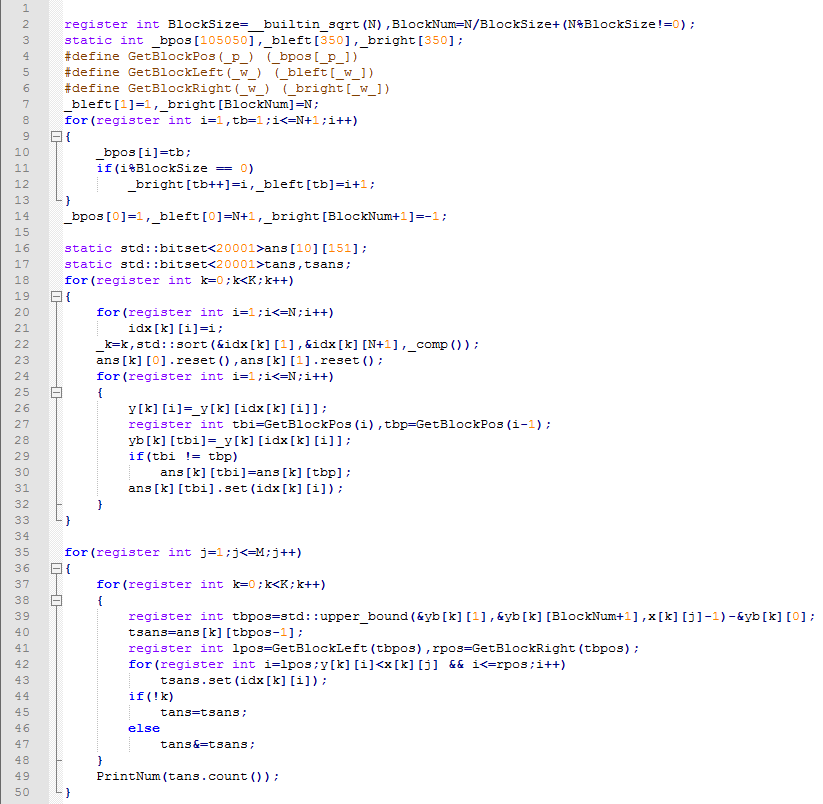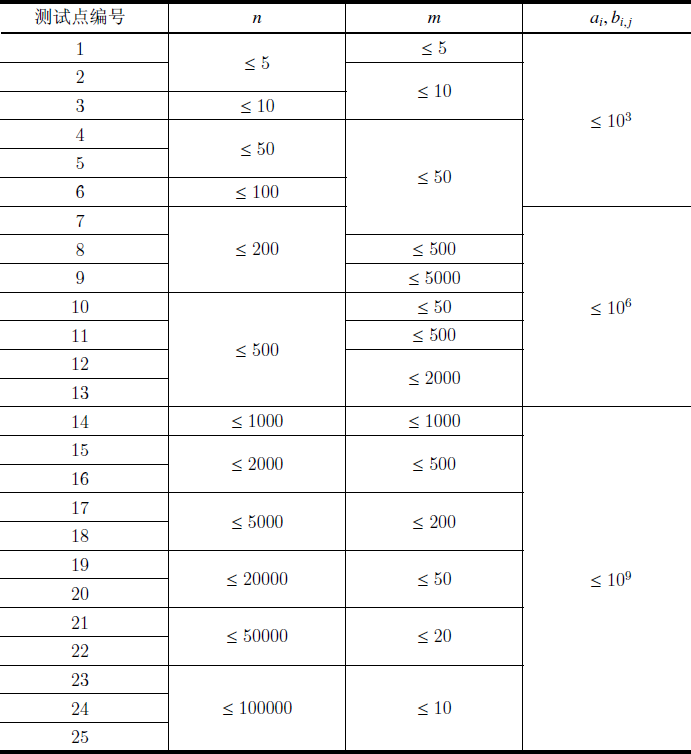# [研学] 偏序问题的研究

• ### 高维偏序…

# [BJOI2018] 链上二次求和

• ### 题目描述

YJC 有一棵 $$n$$ 个节点的树， $$i$$、$$i+1$$ （$$1 \leq i < n$$）节点间有一条边，第 $$i$$ 个点的权值为整数 $$a_i$$ 。

# YZOJ P4611 区间加多项式（YJC 的数组/多项式？）

• ### 题目描述

YJC 在 AK 了一场校内之后，对其中的一题（P2036 「A Simple Data Structure Problem II 」）产生了兴趣。

$$opt=1$$，给定 $$L, R$$，输出 $$\sum\limits_{i=L}^R c_i$$ 的值对 $$998244353$$ 取模后的结果；

$$opt=0$$，给定 $$L, R$$ 以及 $$K$$ 次多项式 $$f(x)=\sum\limits_{k=0}^K a_kx^k$$，对 $$c_L, c_{L+1}, \cdots, c_R$$ 分别加上 $$f(1), f(2), \cdots, f(R-L+1)$$ 的值。

# YZOJ P4637 [CSP-S 2019 五校联训 Round 2]由比滨结衣(sqrt)

• ### 题目描述

1 l r x 将区间 $$[l,r]$$ 中的所有数变为 $$x$$。

2 l r x 查询区间 $$[l,r]$$ 中数字 $$x$$ 的出现次数。

…

# YZOJ P3942 gss2加强版

• ### 题目描述

U x y：将第 $$x$$ 个数修改成 $$y$$ ；

Q x y：计算从第 $$x$$ 个数至第 $$y$$ 个数中不同数的和并输出。如对于一段数 $$1,2,3,2,7$$，它的值是 $$13=1+2+3+7$$ 。

• ### 数据规模与约定

$$n \leq 100000 , m \leq 100000$$

Source： BZOJ 2883…

# YZOJ P2905 [PA2014]Druzyny

• ### 题目描述

OI组的神犇们决定把这题改造一下：

dick32165401：改成只有编号连续的的一段才可以分一组。

runzhe2000：判定可行解可能会被爆搜水过，最大化分的组数就不那么容易水过了。

E.Space：不仅要最大化组数，还要求出最大化组数的方案数。

ct：数据范围就出100万好了。

• ### 样例输出

Source: BZOJ 3711

# [校内训练20161216] T2 版本管理git

• ### 题目描述

$$F$$ 公司有一个项目 $$U$$，这个项目由一个字符串构成。版本 $$0$$ 为空串。有 $$n$$ 个开发者按顺序对这个项目进行了修改，其中第 $$i$$ 个开发者将第 $$p_i ( 0 \le p_i < i)$$ 个版本的开头添加上一个字符 $$c_i (1 \le c_i \le m)$$ 作为新的版本 $$i$$。

• ### 数据规模与约定Source：ARC067 F – Yakiniku